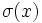Automorphism that preserves conjugacy classes for a generating set

This article defines an automorphism property, viz a property of group automorphisms. Hence, it also defines a function property (property of functions from a group to itself)
View other automorphism properties OR View other function properties

Definition

Suppose$G$ is a group and$\sigma$ is an automorphism of$G$.We say that$\sigma$ is an automorphism that preserves conjugacy classes for a generating set if the folllowing equivalent conditions hold:

1. There is a generating set$S$ for$G$ such that, for all$x \in S$,$\sigma(x)$ is in the conjugacy class of$x$, i.e., there exists$g \in G$ such that$\sigma(x) = gxg^{-1}$. Note that$g$ depends on$x$.
2. There is a symmetric subset$T$ of$G$ that is a generating set of$G$ and such that, for all$x \in T$,$\sigma(x)$ is in the conjugacy class of$x$, i.e., there exists$g \in G$ such that$\sigma(x) = gxg^{-1}$. Note that$g$ depends on$x$.
3. There is a normal subset$N$ of$G$ that is a generating set of$G$ and such that, for all$x \in N$,$\sigma(x)$ is in the conjugacy class of$x$, i.e., there exists$g \in G$ such that$\sigma(x) = gxg^{-1}$. Note that$g$ depends on$x$.

Relation with other properties

Stronger properties

Property Meaning Proof of implication Proof of strictness (reverse implication failure) Intermediate notions
inner automorphism an automorphism for which there exists a single element$g \in G$ such that the automorphism is the map$x \mapsto gxg^{-1}$ (obvious) (via class-preserving automorphism) |FULL LIST, MORE INFO
locally inner automorphism looks like an inner automorphism in its effect on any finite subset (obvious) (via class-preserving automorphism) |FULL LIST, MORE INFO
class-preserving automorphism sends every element to within its conjugacy class (obvious) preserves conjugacy classes for a generating set not implies class-preserving |FULL LIST, MORE INFO

Weaker properties

Property Meaning Proof of implication Proof of strictness (reverse implication failure) Intermediate notions
IA-automorphism sends every element to within its coset of the derived subgroup, or equivalently, induces the identity automorphism on the abelianization preserves conjugacy classes for a generating set implies IA IA-automorphism|automorphism that preserves conjugacy classes for a generating set]]